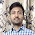## Sunday, August 16, 2015

### Similar and Congruent Triangles their Formulas, Proof and Application in Questions, Basic Proportionality Theorem, Midpoint Theorem

In the previous Video I told that I would be discussing Angle Bisector Theorem in this video lecture but in order to explain you the proof and application of Angle Bisector Theorem it is important that you understand in detail the concept, proof and application of Similar Triangles.

So in this video I have taken in depth discussion of the concept of Congruent Triangles and Similar Triangles. The properties and proof of both of these topics have been explained in full detail and all types of formulas that are derived by their application have been shown in the video. Also how you are going to proceed with questions relating to this topic have also been explained. Two more concepts (BASIC PROPORTIONALITY THEOREM AND MIDPOINT THEOREM) have also been told. Basically these two theorems are manifestation of Similar Triangles so I have taken an integrated approach to make you understand this topic and have explained these concepts while discussing relating to the same. Questions are given below in the snapshot -

1.2.